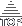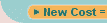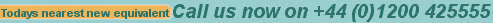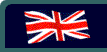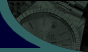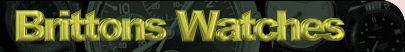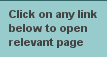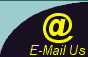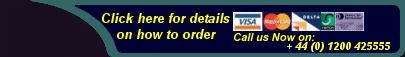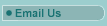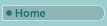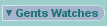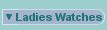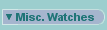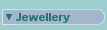Rings Over £100
Rings Under £100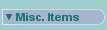Search this site forBulova Serial numbers indicating production dates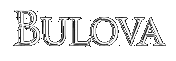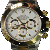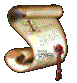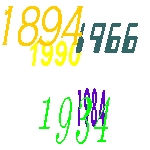Company History

Dating your Accutron is really quite a simple procedure.
Bulova mark there dates using a date code system . This can be found on the back of the case and on the movement. No need to worry about long serial numbers on these!

The code consists of one letter and one number, eg. M7. They used the letter M to signify the decade 1960 to the end of 1969, and the letter N to indicate 1970 to the end of 1979. The digit represents the actual year in that decade. Therefore, M7 is interpreted as 1967.

L=1950s, M=1960s, N=1970s   example: L3=1953, M4=1964, N5=1975, etc.

It is possible that you may have a watch in which the date code on the movement is different to the date code on the case. That is common, however, it has been known to never seen an original watch that had a variation of more than one year. If you see one that has three or more years difference in the date codes, I suggest that the watch has been re-cased or made from 2 separate watches. This probably doesn't really matter unless you are a serious collector and are only interested in completely original items.

The dates codes started in 1948 with"A8" and carried on in sequence with a few exceptions , they missed O out I suppose because of the confusion with zero and then missed "Q,R and S" out for what reason I don't know maybe someone at bulova just didn't like those letters , after 1999 to my knowledge they reverted back to the "A" series numbers again I'm not a 100% percent sure but I had a very modern quartz bulova which clearly beared a date code of "A0" so that's what I'm baseing it on

LIST OF DATE CODES FOUND ON BULOVAS FROM 1948 UNTIL THE MODERN DAY

 A8 = 1948 N5 =1975 A9 = 1949 N6 =1976 L0 = 1950 N7 =1977 L1 =1951 N8 =1978 L2 =1952 N9 =1979 L3 =1953 P0 =1980 L4 =1954 P1 =1981 L5 =1955 P2 =1982 L6 =1956 P3 =1983 L7 =1957 P4 =1984 L8 =1958 P5 =1985 L9 =1959 P6 =1986 M0 =1960 P7 =1987 M1 =1961 P8 =1988 M2 =1962 P9 =1989 M3 =1963 T0 =1990 M4 =1964 T1=1991 M5 =1965 T2 =1992 M6=1966 T3 =1993 M7 =1967 T4 =1994 M8 =1968 T5 =1995 M9 =1969 T6 =1996 N0 =1970 T7 =1997 N1 =1971 T8 =1998 N2 =1972 T9 =1999 N3 =1973 A0 = 2000 N4 =1974 A1 = 2001

 Please remember that this is just an approximate guide and can not be used as a 100% accurate outlook as per how old your watch might be.Should you require a more conclusive valuation of your time piece then please do not hesitate to email us. To determine the approximate age of your watch, the serial number should be located either on the case back or on the inside of the watch stamped oto the movementSimply match up the number on the watch to the nearest number on this page to determine the APPROXIMATE age.

Back to Main Watch Information Index